## Introduction

In many statistical applications, estimating the covariance for a set of random variables is a critical task. The covariance is useful because it characterizes the relationship between variables. For instance, suppose we have three variables $$X, Y, \mbox{ and } Z$$ and their covariance matrix is of the form

$\Sigma_{xyz} = \begin{pmatrix} 1 & 0 & 0.5 \ 0 & 1 & 0 \ 0.5 & 0 & 1 \end{pmatrix}$

We can gather valuable information from this matrix. First of all, we know that each of the variables has an equal variance of 1. Second, we know that variables $$X$$ and $$Y$$ are likely independent because the covariance between the two is equal to 0. This implies that any information in $$X$$ is useless in trying to gather information about $$Y$$. Lastly, we know that variables $$X$$ and $$Z$$ are moderately, positively correlated because their covariance is 0.5.

Unfortunately, estimating $$\Sigma$$ well is often computationally expensive and, in a few settings, extremely challenging. For this reason, emphasis in the literature and elsewhere has been placed on estimating the inverse of $$\Sigma$$ which we like to denote as $$\Omega \equiv \Sigma^{-1}$$.

ADMMsigma is designed to estimate a robust $$\Omega$$ efficiently while also allowing for flexibility and rapid experimentation for the end user.

We will illustrate this with a short simulation.

\vspace{0.5cm}

## Simulation

Let us generate some data.

\vspace{0.5cm}

library(ADMMsigma)

#  generate data from a sparse matrix
# first compute covariance matrix
S = matrix(0.7, nrow = 5, ncol = 5)
for (i in 1:5){
for (j in 1:5){
S[i, j] = S[i, j]^abs(i - j)
}
}

# generate 100 x 5 matrix with rows drawn from iid N_p(0, S)
set.seed(123)
Z = matrix(rnorm(100*5), nrow = 100, ncol = 5)
out = eigen(S, symmetric = TRUE)
S.sqrt = out$vectors %*% diag(out$values^0.5) %*% t(out$vectors) X = Z %*% S.sqrt # snap shot of data head(X)  ## [,1] [,2] [,3] [,4] [,5] ## [1,] -0.4311177 -0.217744186 1.276826576 -0.1061308 -0.02363953 ## [2,] -0.0418538 0.304253474 0.688201742 -0.5976510 -1.06758924 ## [3,] 1.1344174 0.004493877 -0.440059159 -0.9793198 -0.86953222 ## [4,] -0.0738241 -0.286438212 0.009577281 -0.7850619 -0.32351261 ## [5,] -0.2905499 -0.906939891 -0.656034183 -0.4324413 0.28516534 ## [6,] 1.3761967 0.276942730 -0.297518545 -0.2634814 -1.35944340  \vspace{0.5cm} We have generated 100 samples (5 variables) from a normal distribution with mean equal to zero and an oracle covariance matrix $$S$$. \vspace{0.5cm} # print oracle covariance matrix S  ## [,1] [,2] [,3] [,4] [,5] ## [1,] 1.0000 0.700 0.49 0.343 0.2401 ## [2,] 0.7000 1.000 0.70 0.490 0.3430 ## [3,] 0.4900 0.700 1.00 0.700 0.4900 ## [4,] 0.3430 0.490 0.70 1.000 0.7000 ## [5,] 0.2401 0.343 0.49 0.700 1.0000  # print inverse covariance matrix (omega) round(qr.solve(S), 5)  ## [,1] [,2] [,3] [,4] [,5] ## [1,] 1.96078 -1.37255 0.00000 0.00000 0.00000 ## [2,] -1.37255 2.92157 -1.37255 0.00000 0.00000 ## [3,] 0.00000 -1.37255 2.92157 -1.37255 0.00000 ## [4,] 0.00000 0.00000 -1.37255 2.92157 -1.37255 ## [5,] 0.00000 0.00000 0.00000 -1.37255 1.96078  \vspace{0.5cm} It turns out that this particular oracle covariance matrix (tapered matrix) has an inverse - or precision matrix - that is sparse (tri-diagonal). That is, the precision matrix has many zeros. In this particular setting, we could estimate $$\Omega$$ by taking the inverse of the sample covariance matrix $$\hat{S} = \sum_{i = 1}^{n}(X_{i} - \bar{X})(X_{i} - \bar{X})^{T}/n$$: \vspace{0.5cm} # print inverse of sample precision matrix (perhaps a bad estimate) round(qr.solve(cov(X)*(nrow(X) - 1)/nrow(X)), 5)  ## [,1] [,2] [,3] [,4] [,5] ## [1,] 2.32976 -1.55033 0.22105 -0.08607 0.24309 ## [2,] -1.55033 3.27561 -1.68026 -0.14277 0.18949 ## [3,] 0.22105 -1.68026 3.19897 -1.25158 -0.11016 ## [4,] -0.08607 -0.14277 -1.25158 2.76790 -1.37226 ## [5,] 0.24309 0.18949 -0.11016 -1.37226 2.05377  \vspace{0.5cm} However, because $$\Omega$$ is sparse, this estimator will likely perform very poorly. Notice the number of zeros in our oracle precision matrix compared to the inverse of the sample covariance matrix. Instead, we will use ADMMsigma to estimate $$\Omega$$. By default, ADMMsigma will construct $$\Omega$$ using an elastic-net penalty and choose the optimal lam and alpha tuning parameters. \vspace{0.5cm} # elastic-net type penalty (set tolerance to 1e-8) ADMMsigma(X, tol.abs = 1e-8, tol.rel = 1e-8)  ## ## Call: ADMMsigma(X = X, tol.abs = 1e-08, tol.rel = 1e-08) ## ## Iterations: 48 ## ## Tuning parameters: ## log10(lam) alpha ## [1,] -1.599 1 ## ## Log-likelihood: -108.41003 ## ## Omega: ## [,1] [,2] [,3] [,4] [,5] ## [1,] 2.15283 -1.26902 0.00000 0.00000 0.19765 ## [2,] -1.26902 2.79032 -1.32206 -0.08056 0.00925 ## [3,] 0.00000 -1.32206 2.85470 -1.17072 -0.00865 ## [4,] 0.00000 -0.08056 -1.17072 2.49554 -1.18959 ## [5,] 0.19765 0.00925 -0.00865 -1.18959 1.88121  \vspace{0.5cm} We can see that the optimal alpha value selected was 1. This selection corresponds with a lasso penalty – a special case of the elastic-net penalty. Further, a lasso penalty embeds an assumption in the estimate (call it $$\hat{\Omega}$$) that the true $$\Omega$$ is sparse. Thus the package has automatically selected the penalty that most-appropriately matches the true data-generating precision matrix. We can also explicitly assume sparsity in our estimate by restricting the class of penalties to the lasso. We do this by setting alpha = 1 in our function: \vspace{0.5cm} # lasso penalty (default tolerance) ADMMsigma(X, alpha = 1)  ## ## Call: ADMMsigma(X = X, alpha = 1) ## ## Iterations: 24 ## ## Tuning parameters: ## log10(lam) alpha ## [1,] -1.599 1 ## ## Log-likelihood: -108.41193 ## ## Omega: ## [,1] [,2] [,3] [,4] [,5] ## [1,] 2.15308 -1.26962 0.00000 0.00000 0.19733 ## [2,] -1.26962 2.79103 -1.32199 -0.08135 0.00978 ## [3,] 0.00000 -1.32199 2.85361 -1.16953 -0.00921 ## [4,] 0.00000 -0.08135 -1.16953 2.49459 -1.18914 ## [5,] 0.19733 0.00978 -0.00921 -1.18914 1.88096  \vspace{0.5cm} We might also want to restrict alpha = 0.5: \vspace{0.5cm} # elastic-net penalty (alpha = 0.5) ADMMsigma(X, alpha = 0.5)  ## ## Call: ADMMsigma(X = X, alpha = 0.5) ## ## Iterations: 20 ## ## Tuning parameters: ## log10(lam) alpha ## [1,] -1.821 0.5 ## ## Log-likelihood: -101.13591 ## ## Omega: ## [,1] [,2] [,3] [,4] [,5] ## [1,] 2.20055 -1.32510 0.01689 -0.00350 0.21805 ## [2,] -1.32510 2.90739 -1.37666 -0.19054 0.13642 ## [3,] 0.01689 -1.37666 2.92556 -1.12877 -0.12032 ## [4,] -0.00350 -0.19054 -1.12877 2.56559 -1.23466 ## [5,] 0.21805 0.13642 -0.12032 -1.23466 1.94525  \newpage Or maybe we want to assume that $$\Omega$$ is not sparse but has entries close to zero. In this case, a ridge penalty would be most appropriate. We can estimate an $$\Omega$$ of this form by setting alpha = 0 in the ADMMsigma function or using the RIDGEsigma function. RIDGEsigma uses a closed-form solution rather than an algorithm to compute its estimate – and for this reason should be preferred in most cases (less computationally intensive). \vspace{0.5cm} # ridge penalty ADMMsigma(X, alpha = 0)  ## ## Call: ADMMsigma(X = X, alpha = 0) ## ## Iterations: 31 ## ## Tuning parameters: ## log10(lam) alpha ## [1,] -1.821 0 ## ## Log-likelihood: -99.19745 ## ## Omega: ## [,1] [,2] [,3] [,4] [,5] ## [1,] 2.18987 -1.31545 0.04524 -0.04093 0.23512 ## [2,] -1.31545 2.90045 -1.37070 -0.22626 0.17807 ## [3,] 0.04524 -1.37070 2.89459 -1.07653 -0.17369 ## [4,] -0.04093 -0.22626 -1.07653 2.55028 -1.22785 ## [5,] 0.23512 0.17807 -0.17369 -1.22785 1.95494  # ridge penalty (using closed-form solution) RIDGEsigma(X, lam = 10^seq(-8, 8, 0.01))  ## ## Call: RIDGEsigma(X = X, lam = 10^seq(-8, 8, 0.01)) ## ## Tuning parameter: ## log10(lam) lam ## [1,] -2.17 0.007 ## ## Log-likelihood: -109.18156 ## ## Omega: ## [,1] [,2] [,3] [,4] [,5] ## [1,] 2.15416 -1.31185 0.08499 -0.05571 0.22862 ## [2,] -1.31185 2.85605 -1.36677 -0.19650 0.16880 ## [3,] 0.08499 -1.36677 2.82606 -1.06325 -0.14946 ## [4,] -0.05571 -0.19650 -1.06325 2.50721 -1.21935 ## [5,] 0.22862 0.16880 -0.14946 -1.21935 1.92871  \newpage ADMMsigma also has the capability to provide plots for the cross validation errors. This allows the user to analyze and select the appropriate tuning parameters. In the heatmap plot below, the more bright (white) areas of the heat map correspond to a better tuning parameter selection. \vspace{0.5cm} # produce CV heat map for ADMMsigma ADMM = ADMMsigma(X, tol.abs = 1e-8, tol.rel = 1e-8) plot(ADMM, type = "heatmap")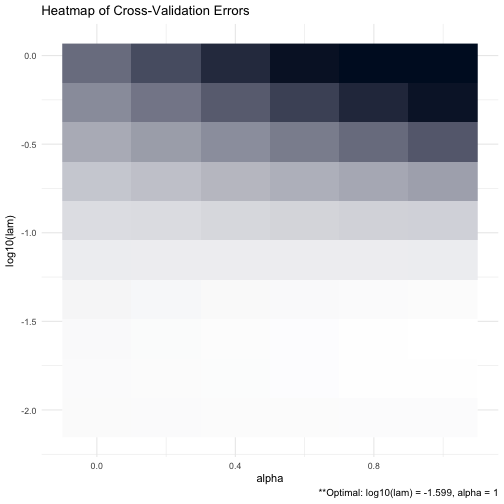\vspace{0.5cm} We can also produce a line graph of the cross validation errors: \vspace{0.5cm} # produce line graph for CV errors for ADMMsigma plot(ADMM, type = "line")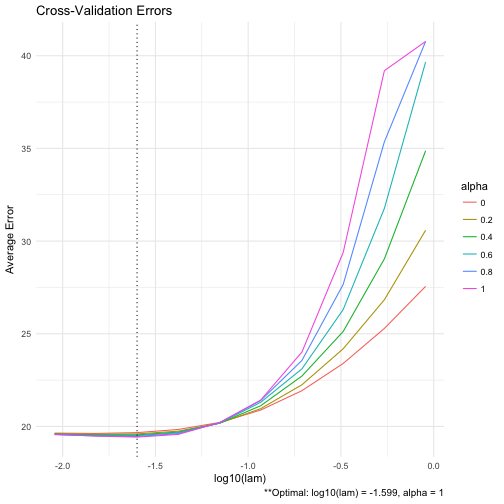\vspace{0.5cm} And similarly for RIDGEsigma: \vspace{0.5cm} # produce CV heat map for RIDGEsigma RIDGE = RIDGEsigma(X, lam = 10^seq(-8, 8, 0.01)) plot(RIDGE, type = "heatmap")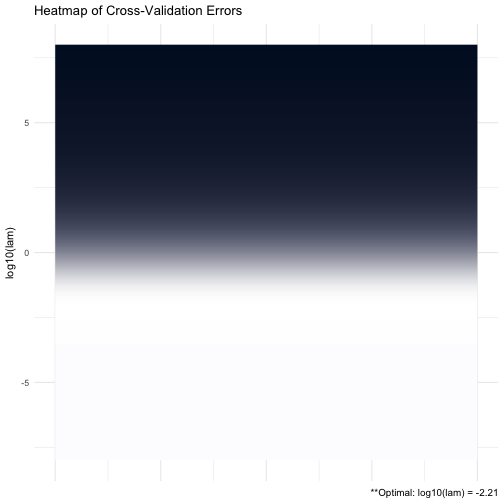# produce line graph for CV errors for RIDGEsigma plot(RIDGE, type = "line")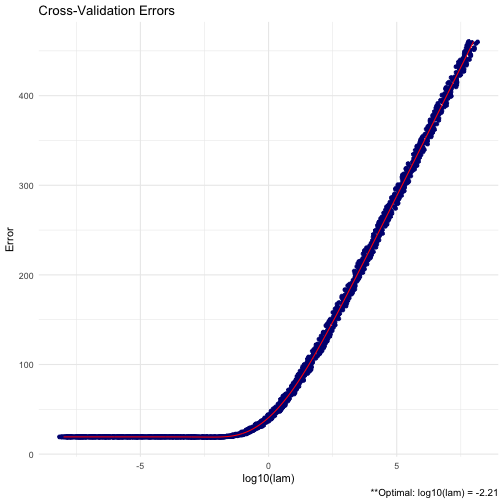\vspace{0.5cm} ## More advanced options ADMMsigma contains a number of different criteria for selecting the optimal tuning parameters during cross validation. The package default is to choose the tuning parameters that maximize the log-likelihood (crit.cv = loglik). Other options include AIC and BIC. \vspace{0.5cm} # AIC plot(ADMMsigma(X, crit.cv = "AIC"))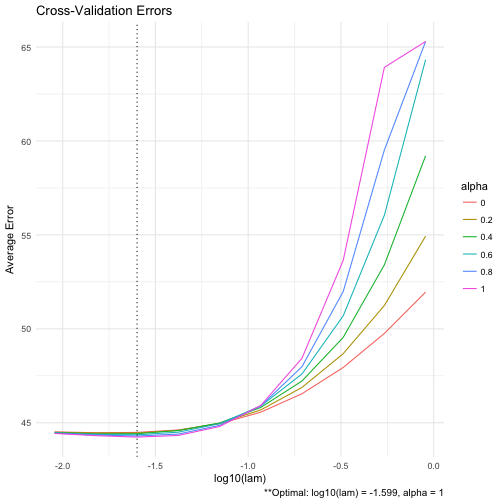# BIC plot(ADMMsigma(X, crit.cv = "BIC"))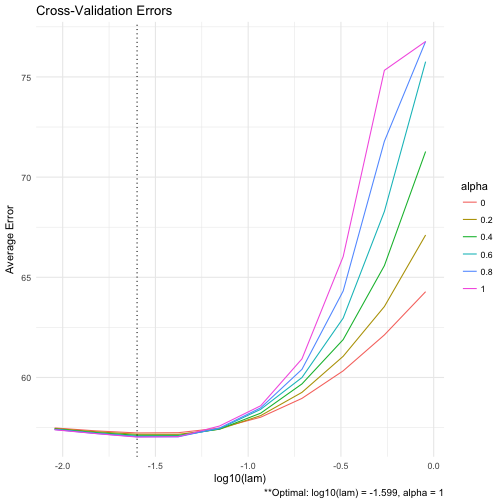\vspace{0.5cm} This allows the user to select appropriate tuning parameters under various decision criteria. We also have the option to print all of the estimated precision matrices for each tuning parameter combination using the path option. This option should be used with extreme care when the dimension and sample size is large – you may run into memory issues. \vspace{0.5cm} # keep all estimates using path ADMM = ADMMsigma(X, path = TRUE) # print only first three objects ADMM$Path[,,1:3]

## , , 1
##
##             [,1]       [,2]        [,3]        [,4]       [,5]
## [1,]  2.23564293 -1.3907385  0.09733949 -0.05164777  0.2371902
## [2,] -1.39073853  3.0203360 -1.46649296 -0.20515964  0.1853698
## [3,]  0.09733949 -1.4664930  2.99143190 -1.13222767 -0.1546670
## [4,] -0.05164777 -0.2051596 -1.13222767  2.62529228 -1.2784837
## [5,]  0.23719016  0.1853698 -0.15466701 -1.27848372  1.9904020
##
## , , 2
##
##             [,1]       [,2]        [,3]        [,4]       [,5]
## [1,]  2.23931273 -1.3958729  0.09291678 -0.04294401  0.2328060
## [2,] -1.39587292  3.0268186 -1.47191266 -0.19541851  0.1752293
## [3,]  0.09291678 -1.4719127  3.00111254 -1.14521358 -0.1414309
## [4,] -0.04294401 -0.1954185 -1.14521358  2.62901503 -1.2805458
## [5,]  0.23280604  0.1752293 -0.14143095 -1.28054584  1.9883394
##
## , , 3
##
##             [,1]       [,2]        [,3]        [,4]       [,5]
## [1,]  2.24280962 -1.4002743  0.08791665 -0.03439921  0.2287425
## [2,] -1.40027427  3.0316785 -1.47634387 -0.18487822  0.1641385
## [3,]  0.08791665 -1.4763439  3.01134698 -1.16017964 -0.1269540
## [4,] -0.03439921 -0.1848782 -1.16017964  2.63419383 -1.2829947
## [5,]  0.22874252  0.1641385 -0.12695399 -1.28299472  1.9861783


\vspace{0.5cm}

A huge issue in precision matrix estimation is the computational complexity when the sample size and dimension of our data is particularly large. There are a number of built-in options in ADMMsigma that can be used to improve computation speed:

• Reduce the number of lam values during cross validation. The default number is 10.

\vspace{0.5cm}

# reduce number of lam to 5


\vspace{0.5cm}

• Reduce the number of K folds during cross validation. The default number is 5.

\vspace{0.5cm}

# reduce number of folds to 3


\vspace{0.5cm}

• Relax the convergence critera for the ADMM algorithm using the tol.abs and tol.rel options. The default for each is 1e-4.

\vspace{0.5cm}

# relax convergence criteria


\vspace{0.5cm}

• Adjust the maximum number of iterations. The default is 1e4.

\vspace{0.5cm}

# adjust maximum number of iterations


\vspace{0.5cm}

• Adjust adjmaxit. This allows the user to adjust the maximum number of iterations after the first lam tuning parameter has fully converged during cross validation. This allows for one-step estimators and can greatly reduce the time required for the cross validation procedure while still choosing near-optimal tuning parameters.

\vspace{0.5cm}

# adjust adjmaxit


\vspace{0.5cm}

• We can also opt to run our cross validation procedure in parallel. The user should check how many cores are on their system before using this option

\vspace{0.5cm}

# parallel CV